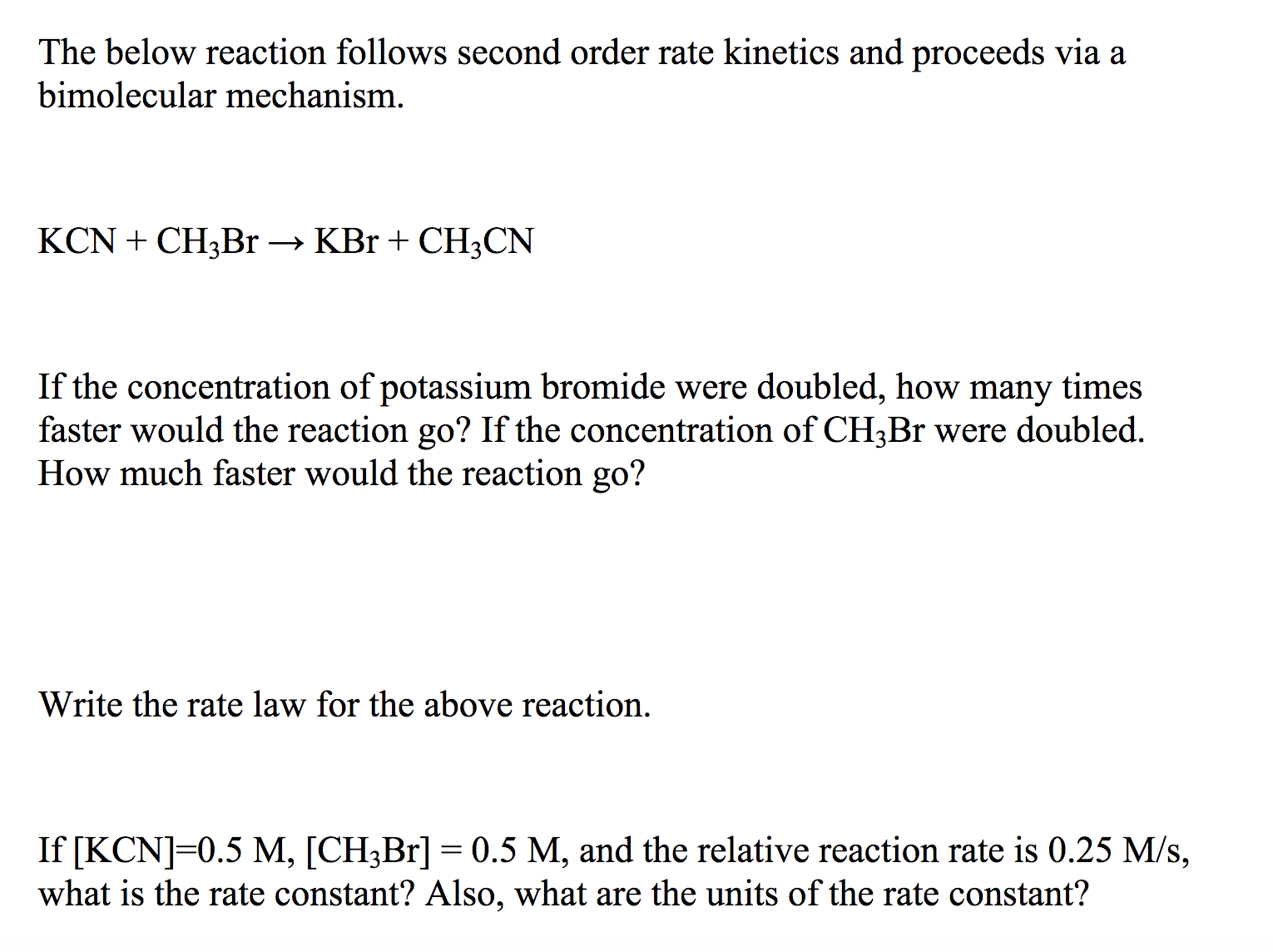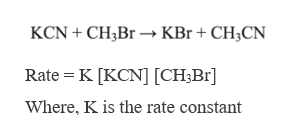The below reaction follows second order rate kinetics and proceeds via abimolecular mechanism.KCNCH3B1 -» KBr + CH3CNIf the concentration of potassium bromide were doubled, how many timesfaster would the reaction go? If the concentration of CH3Br were doubled.How much faster would the reaction go?Write the rate law for the above reaction.If [KCN] 0.5 M, [CH3Br] 0.5 M, and the relative reaction rate is 0.25 M/s,what is the rate constant? Also, what are the units of the rate constant?

Questionhelp_outlineImage TranscriptioncloseThe below reaction follows second order rate kinetics and proceeds via a bimolecular mechanism. KCNCH3B1 -» KBr + CH3CN If the concentration of potassium bromide were doubled, how many times faster would the reaction go? If the concentration of CH3Br were doubled. How much faster would the reaction go? Write the rate law for the above reaction. If [KCN] 0.5 M, [CH3Br] 0.5 M, and the relative reaction rate is 0.25 M/s, what is the rate constant? Also, what are the units of the rate constant? fullscreen
Step 1

The rate law of the given reaction can be written as,help_outlineImage TranscriptioncloseKCN CH3Br» KBr + CH3CN Rate K [KCN] [CH3Br Where, K is the rate constant fullscreen
Step 2

If the concentration of KBr is doubled then it would not affect the rate of reaction. The reaction will occur at the same rate as the rate does not depends on the concentration of the products. It only depends on the concentration of the reactant and here KBr is a product. Hence no change in the speed of reaction.

Step 3

If the concentration of CH3Br is doubled then, the rate of reaction will also double as per the rate equation mentioned above. Since CH3Br is a reactant and the rate...

Want to see the full answer?

See Solution

Want to see this answer and more?

Our solutions are written by experts, many with advanced degrees, and available 24/7

See Solution
Tagged in

Chemical Kinetics